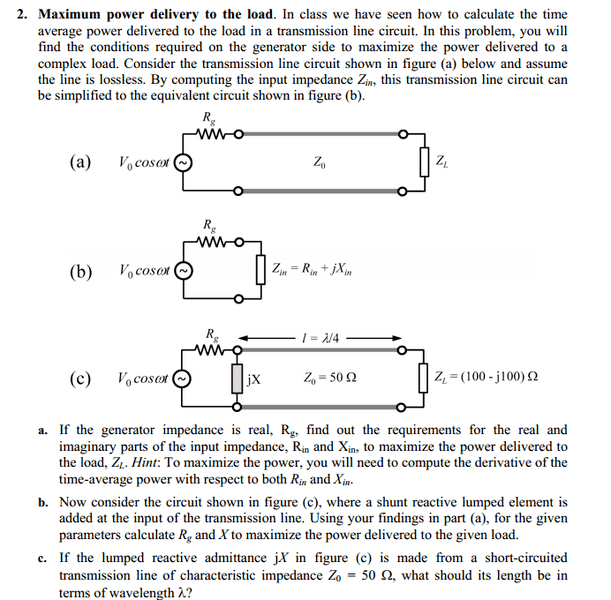# Maximze power delivered to load (lossless tranmsion line)

• nagr

## Homework Statement## Homework Equations

Time Avg. Power = $\frac{|V0+|^2}{2*Z0}$ [1-|$\Gamma|^2$]
for line of l=$\frac{λ}{4}$+n$\frac{λ}{2}$ (where n=0 here), Zin=$\frac{Z0^2}{ZL}$

## The Attempt at a Solution

Bit confused what to do in the 1st part? I know that max. power is delivered to load when Z0=ZL because then there will be no reflection, so |$\Gamma$|=0 which is obvious from the equation that power is maximized (also my professor explained this). But to prove this, am i expected to take the derivative of the equation for time avg. power, with respect to Z0=Zin=R+jX? I am unsure how to do this, first of all. Also here in this problem (c) Z0 is not same as ZL so power delivered is not maximized. But using the equation above I calculated Zin=12.5+j12.5 from which I found X=12.5 and Rg i assumed was the real part of Zin=12.5. i was wondering though if I did this right so far.

Last edited:

1. Compute Zin which will be a function of Z0 and θ of the line, and ZL. θ = βx, β = ω(LC)(1/2), and x = physical length of line. See illustration (b).

2. Compute V across Zin. You can assume Vin = 1.

3. Compute I.

4. Compute power P = |V||I|cos(ψ) or Re{VI*}.

5. Find Rin and Xin which maximize P.

V and I are phasors.

the general equation for Zin in my book is Zin=Z0($\frac{zL+j(tan(βl)))}{1+j(zL*tan(βl))}$) where zL=$\frac{ZL}{Z0}$ but i don't think this helps me much. for problem (b), this is what I did so far: since length of line is a special case l=$\frac{λ}{4}$, i used the equation for Zin I listed and got Zin=12.5+j12.5. so according to figure (b), I thught this would mean Zin in parallel with the shunt element jX, I found the value for X so that the imaginary part would cancel out, so X=-25. this would mean Rg=(Zin||-j25) for maximum power delivery so Rg=25.

Last edited:
ok, my professor explained this to me so obviously i was doing this wrong. If I use the equation he gave me, Pavg=$\frac{1}{2}$Re(V x I*)=$\frac{1}{2}$ Re[$\frac{Vg}{Zg+(Rin+Xin)}$((Rin+Xin)($\frac{Vg}{Zg+(Rin-Xin}))$] where i substituted Zin=Rin+Xin and use Vg=Vo and I ended up with 1/2 Re[$\frac{Vo^2Rin+jVo^2Xin}{(Rg+Rin)^2+X^2}$] but I am not very good with math :P so i don't know if i did this right. would appreciate if you could help me out.